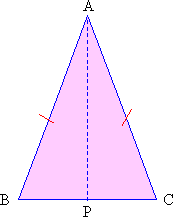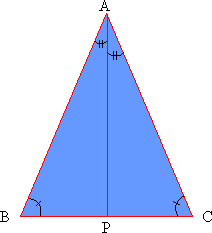Home MonkeyNotes Printable Notes Digital Library Study Guides Message Boards Study Smart Parents Tips College Planning Test Prep Fun Zone Help / FAQ How to Cite New Title Request

 2.6 Sides opposite congruent angles Theorem : If two sides of a triangle are equal, then the angles opposite them are also equal. This can be proven as follows : Consider a D ABC where AB = AC ( figure 2.23 ).Figure 2.23 Proof : To prove m Ð B = m Ð C drop a median from A to BC at point P. Since AP is the median, BP = CP. \ In D ABP and D ACP seg. AB @ seg. AC( given ) seg. BP @ seg. CP( P is midpoint ) seg. AP @ seg. AP( same line ) Therefore the two triangles are congruent by SSS postulate. D ABP @ D ACP \ m Ð B = m Ð C as they are corresponding angles of congruent triangles. The converse of this theorem is also true and can be proven quite easily. Consider D ABC where m Ð B = m Ð C ( figure 2.24 )Figure 2.24 To prove AB = AC drop an angle bisector AP on to BC. Since AP is a bisector m Ð BAP = m Ð CAP m Ð ABP = m Ð ACP ( given ) seg. AP @ seg. AP (same side ) \ D ABP @ D ACP by AAS postulate. Therefore the corresponding sides are equal. \ seg. AB = seg. AC Conclusion :If the two angles of a triangle are equal, then the sides opposite to them are also equal.   ********** Index 2.1 Introduction 2.2 Sum Of The Angles Of A Triangle 2.3 Types of Triangles 2.4 Altitude, Median And Angle Bisector 2.5 Congruence Of Triangles 2.6 Sides Opposite Congruent Angles Chapter 3
 Search: All Products Books Popular Music Classical Music Video DVD Toys & Games Electronics Software Tools & Hardware Outdoor Living Kitchen & Housewares Camera & Photo Cell Phones Keywords: